# TSA Welch VI

LabVIEW 2014 Advanced Signal Processing Toolkit Help

Edition Date: June 2014

Part Number: 372656C-01

»View Product InfoDownload Help (Windows Only)

Owning Palette: Correlation and Spectral Analysis VIs

Computes the single-sided power spectral density (PSD) of a univariate time series using the Welch method, which is a variation of the periodogram method. This VI estimates the PSD by averaging periodograms of overlapping, windowed subsequences of the time series. Wire data to the Xt input to determine the polymorphic instance to use or manually select the instance.

Use the pull-down menu to select an instance of this VI.

 Select an instance TSA Welch (Waveform)TSA Welch (Array)

## TSA Welch (Waveform)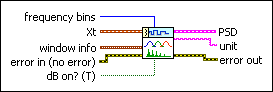frequency bins specifies the number of frequency bins for which this VI computes the single-sided power spectral density PSD. The length of the single-sided PSD is (frequency bins/2+1). The default is 1024.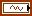Xt specifies the univariate time series.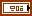window info specifies the information of the sliding window that divides the time series into subsequences.window specifies the time-domain window applied to the time series. Options include None (default), Hanning, Hamming, Blackman-Harris, Exact Blackman, Blackman, Flat Top, 4 Term B-Harris, 7 Term B-Harris, and Low Sidelobe.length specifies the length of the window. A large window generates a power spectral density (PSD) with small bias but results in a coarse PSD plot. A small window generates a smooth PSD plot but leads to large bias. The default is –1, which indicates that the window length equals the length of the input time series.overlap specifies the overlap, in percentage, of the moving window that this VI applies to the time series. This parameter determines how much data this VI reuses for the signal space matrix. A large overlap reduces the variance of the resulting power spectrum but increases computation time. The default is 50, which specifies that the overlap is half of the window length.error in describes error conditions that occur before this node runs. This input provides standard error in functionality.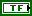dB on? specifies whether this VI returns the PSD in decibels or in a linear scale. If dB on? is TRUE, this VI returns the PSD in decibels. If dB on? is FALSE, this VI returns the PSD in a linear scale. The default is TRUE.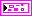PSD returns information about the single-sided power spectral density (PSD).f0 returns the lower boundary, in hertz, of the frequency range.df returns the frequency increment, in hertz.S(f) returns the magnitude of the PSD at each frequency. The value of dB on? determines the unit of measurement for this parameter.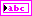unit returns the engineering unit of the PSD. You can specify an engineering unit for a time series by using the TSA Scale to EU VI.error out contains error information. This output provides standard error out functionality.

## TSA Welch (Array)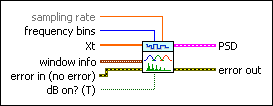sampling rate specifies the sampling rate, in hertz, of the univariate time series Xt. The default is 1.frequency bins specifies the number of frequency bins for which this VI computes the single-sided power spectral density PSD. The length of the single-sided PSD is (frequency bins/2+1). The default is 1024.Xt specifies the univariate time series.window info specifies the information of the sliding window that divides the time series into subsequences.window specifies the time-domain window applied to the time series. Options include None (default), Hanning, Hamming, Blackman-Harris, Exact Blackman, Blackman, Flat Top, 4 Term B-Harris, 7 Term B-Harris, and Low Sidelobe.length specifies the length of the window. A large window generates a power spectral density (PSD) with small bias but results in a coarse PSD plot. A small window generates a smooth PSD plot but leads to large bias. The default is –1, which indicates that the window length equals the length of the input time series.overlap specifies the overlap, in percentage, of the moving window that this VI applies to the time series. This parameter determines how much data this VI reuses for the signal space matrix. A large overlap reduces the variance of the resulting power spectrum but increases computation time. The default is 50, which specifies that the overlap is half of the window length.error in describes error conditions that occur before this node runs. This input provides standard error in functionality.dB on? specifies whether this VI returns the PSD in decibels or in a linear scale. If dB on? is TRUE, this VI returns the PSD in decibels. If dB on? is FALSE, this VI returns the PSD in a linear scale. The default is TRUE.PSD returns information about the single-sided power spectral density (PSD).f0 returns the lower boundary, in hertz, of the frequency range.df returns the frequency increment, in hertz.S(f) returns the magnitude of the PSD at each frequency. The value of dB on? determines the unit of measurement for this parameter.error out contains error information. This output provides standard error out functionality.

## TSA Welch Details

This VI estimates the PSD of a time series with the Welch method according to the following steps:

1. Divides the time series into subsequences. The size of each subsequence equals the value of the length parameter.
2. Applies a window to each subsequence and computes the PSD of each subsequence with the periodogram method.
3. Averages the PSDs of the subsequences to form the resulting PSD s(f).

The PSD generated with the Welch method has smaller variance and is smoother than the PSD generated with the periodogram method.

## Example

Refer to the Power Spectral Density Estimation VI in the labview\examples\Time Series Analysis\TSAGettingStarted directory for an example of using the TSA Welch VI.